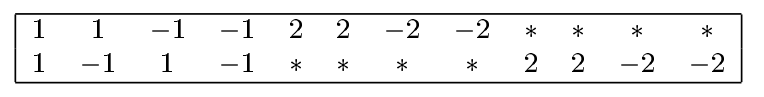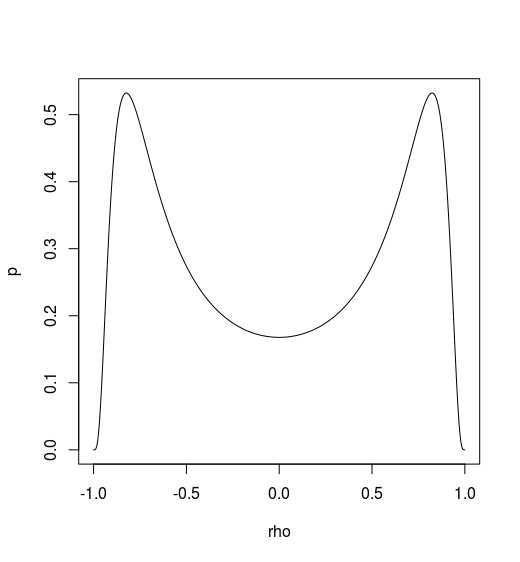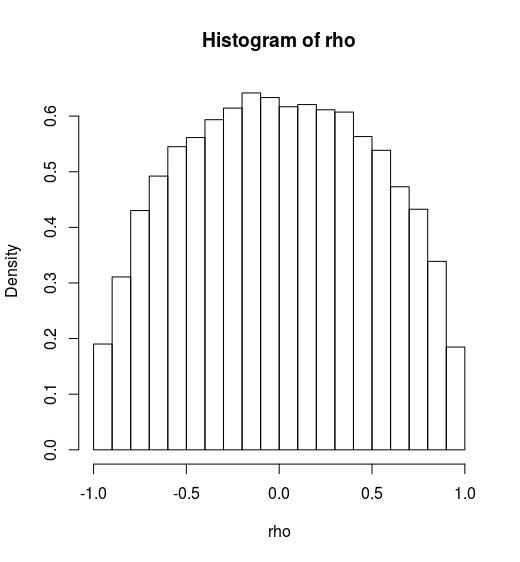# A Bayesian Missing Data Problem

##### Posted on Jul 18, 20170 Comments
Tags: Monte Carlo

## Problem

There is an artificial dataset of bivariate Gaussian observation with missing parts. The symbol * indicates that the value is missing.Denote these data as $y_1,\ldots, y_{12}$, where $y_t=(y_{t,1}, y_{t,2}), t=1,\ldots, 12$. The posterior distribution of $\rho$ given the incomplete data is of interest.

Firstly, the covariance matrix of the bivariate normal is assigned the Jeffrey’s noinformative prior distribution

$\pi(\Sigma) \propto \vert \Sigma\vert^{-\frac{d+1}{2}}$

where $d$ is the dimensionality and equals to 2 in this problem. The posterior distribution of $\Sigma$ given $t$ i.i.d. observed complete data $y_1,\ldots, y_t$ is

$p(\Sigma \mid y_1, \ldots, y_t) \propto \vert\Sigma \vert^{-\frac{d+1+t}{2}}exp\{-\frac{1}{2}tr[\Sigma^{-1} \cdot S]\}$

where $S = (s_{ij})_{22}, s_{ij}=\sum_{s=1}^t y_{s,i}y_{s,j}$.

By letting ${\cal Z}=\Sigma^{-1}$, then ${\cal Z}\sim Wishart(t, S)$.

For this problem,

$p(\Sigma, \mathbf y_{mis}\mid \mathbf y_{obs}) \propto \vert\Sigma \vert^{-\frac{2+1+12}{2}}exp\{-\frac{1}{2}tr[\Sigma^{-1} \cdot S(\mathbf y_{mis})]\}$

where $S(\mathbf y_{mis})$ emphasizes that its value depends on the value of $\mathbf y_{mis}$. We can obtain the posterior distribution of $\Sigma$ by integrating out $\mathbf y_{mis}$. An importance sampling algorithm for achieving this goal can be implemented as follows:

1. Sample $\Sigma$ from some trial distribution $g_0(\Sigma)$
2. Draw $\mathbf y_{mis}$ from $p(\mathbf y_{mis}\mid \mathbf y_{obs},\Sigma)$

It is obvious that the predictive distribution of $\mathbf y_{mis}$ is simply the Gaussian distribution given $\Sigma$. So the key question is how to draw $\Sigma$. A simple idea is to draw $\Sigma$ from its posterior distribution conditional only on the first four complete observations:

$g_0(\Sigma) \propto \vert \Sigma\vert^{-7/2}exp(-tr[\Sigma^{-1}S_4]/2)$

where $S_4$ is the sum of the square matrix computed from the first four observations.

## Implement

The analytical form isHowever, there might be some wrong in my code. You can find my code in Github.If $X_1,\ldots, X_n; X_i\in R^p$ are $n$ independent multivariate Gaussians with mean $\mathbf 0$ and covariance matrix $\Sigma$, the distribution of $S = X’X\sim \cal W_p(\Sigma, n)$, and $S^{-1}\sim W_p^{-1}(\Sigma^{-1}, n)$, where $X = (X_1, X_2,\ldots, X_n)’$.

Liu, Jun S. Monte Carlo strategies in scientific computing. Springer Science & Business Media, 2008.